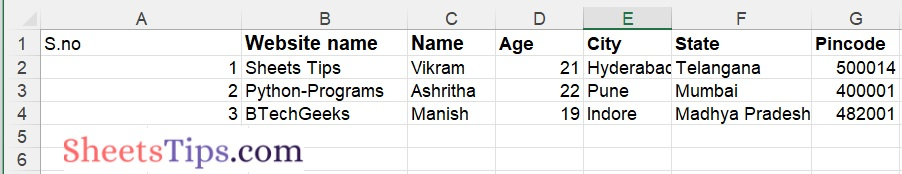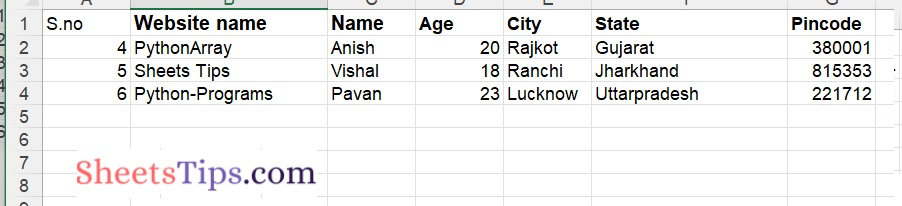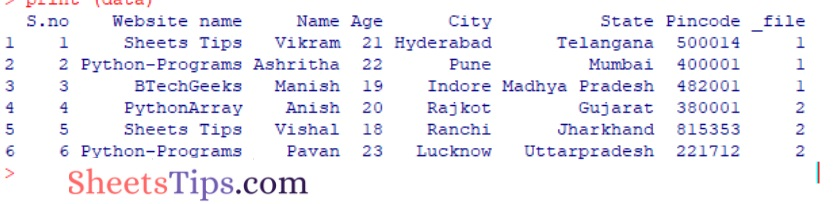# R Program to Combine Multiple Excel Worksheets into Single Dataframe

In this post, let us look at how to use the R language to combine several Excel spreadsheets into a single dataframe.

Here we now work on the following Excel spreadsheet shown in the image below:

SampleExcelFile:

Sheet 1:Sheet 2:## R Program to Combine Multiple Excel Worksheets into Single Dataframe

This can be done using the following ways:

### Method #1: Using the readxl package

The readxl package in R is used to import and read Excel workbooks, allowing you to quickly work with and modify.xslsx sheets. The following syntax can be used to install and load it into the R working environment.

Installation:

`install.packages("readxl")`

### Functions Used:

1)lappy() method:

The lapply() method in R is used to apply a user-defined or pre-defined function to a set of components included within an R list or data frame. The method lapply() returns an object with the same length as the supplied/given object.

Syntax:

`lapply( object, FUNCTION)`

Parameters

object: It is the object to which the function will be applied.

FUNCTION: It is the function that will be applied to different components of the object.

2)excel_sheets() Function:

The excel_sheets() method is initially called to retrieve all of the worksheet names contained in the Excel workbook with the provided file path.

The FUNCTION here is the read_excel method of this package store, which reads the contents of the specified sheet name into a tibble, which is a tabular-like structure used to store data in fixed rows and columns.

The read_excel method is applied to all sheets of the workbook by the lapply() method.

Syntax:

`read_excel(path, sheet)`

Parameters

path: It is the path of the file given as input.

sheet: It is the sheet name to be read.

The final tibble is generated by the built-in method bind_rows(), which takes the individual dataframes as input and assigns them a single ID attribute key. If a column exists in any of the inputs, the result of bind rows() will include that column. The output tibble comprises all of the records from all of the sheets, along with their data types. The column name “Sheet” is used to construct a primary column that leads all of the rows.

### CODE

Approach:

• Import the readxl package using the library() function
• Import thetidyverse package using the library() function
• Give the Path of the folder where Excel files are present as static input and store it in a variable
• Pass the above folder path as an argument to the setwd() function to set the working directory to the above path.
• Pass the file name as an argument to the excel_sheets function of the readxl library to access all the sheets of the excel file and store it in a variable.
• Get the dataframe of the above excel file using the lapply() function
• Combilne all dataframes or sheets of the excel file together using the bind_rows() function by passing the above dataframe, id=”Sheet” as arguments to it.
• Store it in the same variable.
• Print the dataframe values i.e data from all the sheets after combining them using the print() function.
• The Exit of the Program.

Below is the implementation:

```# Import the readxl package using the library() function
# Import thetidyverse package using the library() function
library(tidyverse)

# Give the Path of the folder where Excel files are present as static input
# and store it in a variable

# Pass the above folder path as an argument to the setwd() function to
# set the working directory to the above path.
setwd(folder_path)

# Pass the file name as an argument to the excel_sheets function of the readxl
# library to access all the sheets of the excel file and store it in a variable.
sheet= excel_sheets("sampleExcelFile.xlsx")

# Get the dataframe of the above excel file using the lapply() function
datafrme = lapply(setNames(sheet, sheet),

# Combilne all dataframes or sheets of the excel file together using the bind_rows() function
# by passing the above dataframe, id="Sheet" as arguments to it.
# Store it in the same variable
datafrme = bind_rows(datafrme, .id="Sheet")

# Print the dataframe values i.e data from all the sheets after combining them
# using the print() function
print (datafrme)```

Output:### Method #2: Using the rio package

The rio package is used to support quick and easy data import and export activities in R. Rio deduces information about the file format itself that can be utilized to conveniently read files.

Installation:

`install.packages("rio")`

### Functions Used:

The R language import() and export() functions identify the data structure of a file extension. The import_list() method loads/imports a list of data frames from a multi-object file, such as an Excel workbook or an R zipped file.

Syntax:

`import_list(file,  rbind = FALSE)`

Parameters

file: It is the file name/path of the Excel workbook to work with given as input.

rbind: It represents whether the dataframes should be combined into a single dataframe or Not.

### CODE

Approach:

• Give the Excel file path as static input and store it in a variable
• Importing rio package using the library() function
• Pass the above given excel file, rbind = True as arguments() to the import_list() function to get list of data frames/data from the given excel file and store it in another variable
• Here rbind=TRUE combines the data of all the sheets in an excel file
• Print combined sheets data of the given excel using the print() function.
• The Exit of the Program.

Below is the implementation:

```# Give the Excel file path as static input and store it in a variable

# Importing rio package using the library() function
library(rio)

# Pass the above given excel file, rbind = True as arguments() to the import_list()
# function to get list of data frames/data from the given excel file
# and store it in another variable
# Here rbind=TRUE combines the data of all the sheets in an excel file
combined_sheetsdata <- import_list(Excelfilepath , rbind=TRUE)

# Print combined sheets data of the given excel using the print() function.
print (combined_sheetsdata)
```

Output: